# Multiplying Brackets

## Method 1 -  The arrows method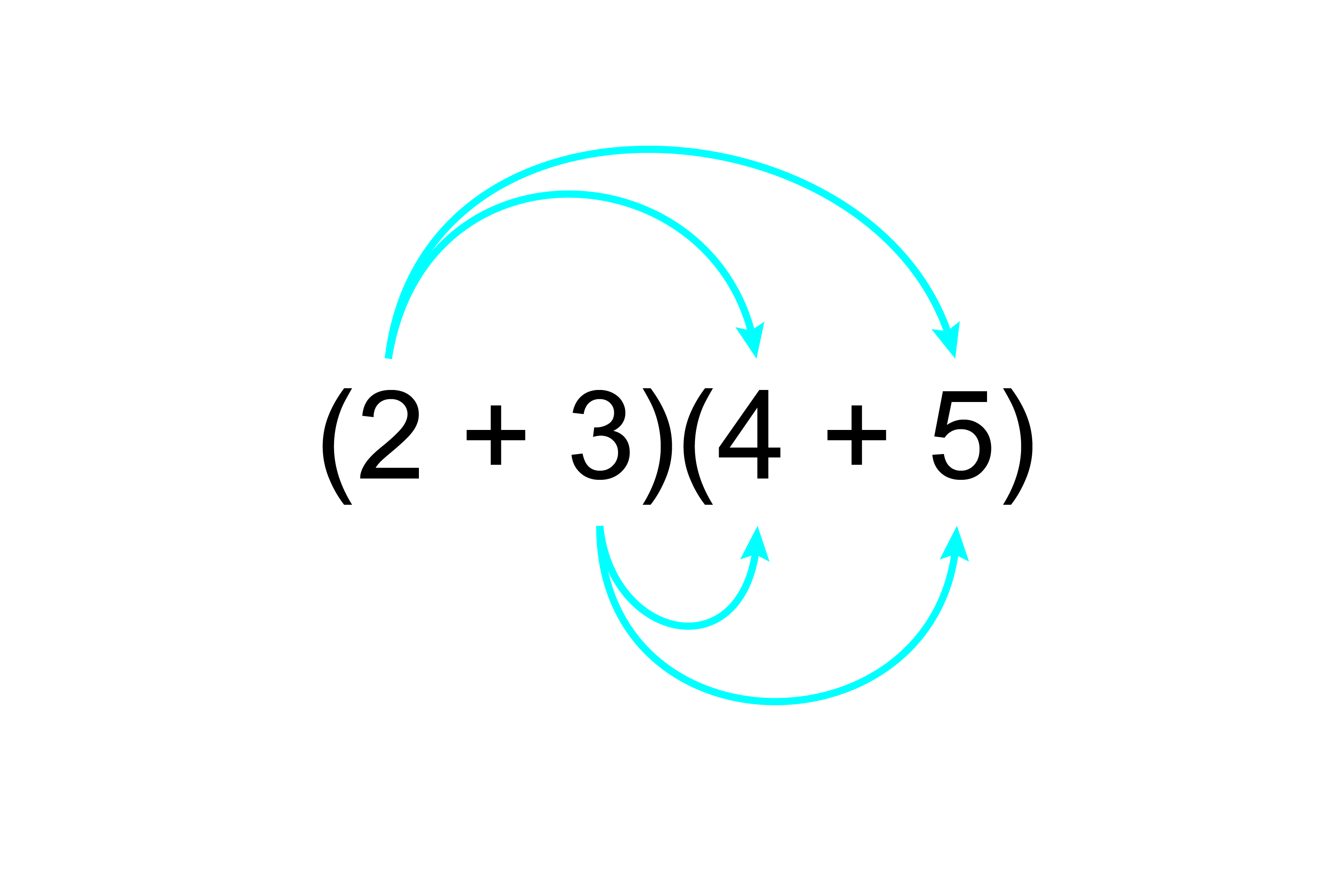2times4+2xx5+3xx4+3xx5

=8+10+12+15

=45

## Method 2 – The woman with the big nose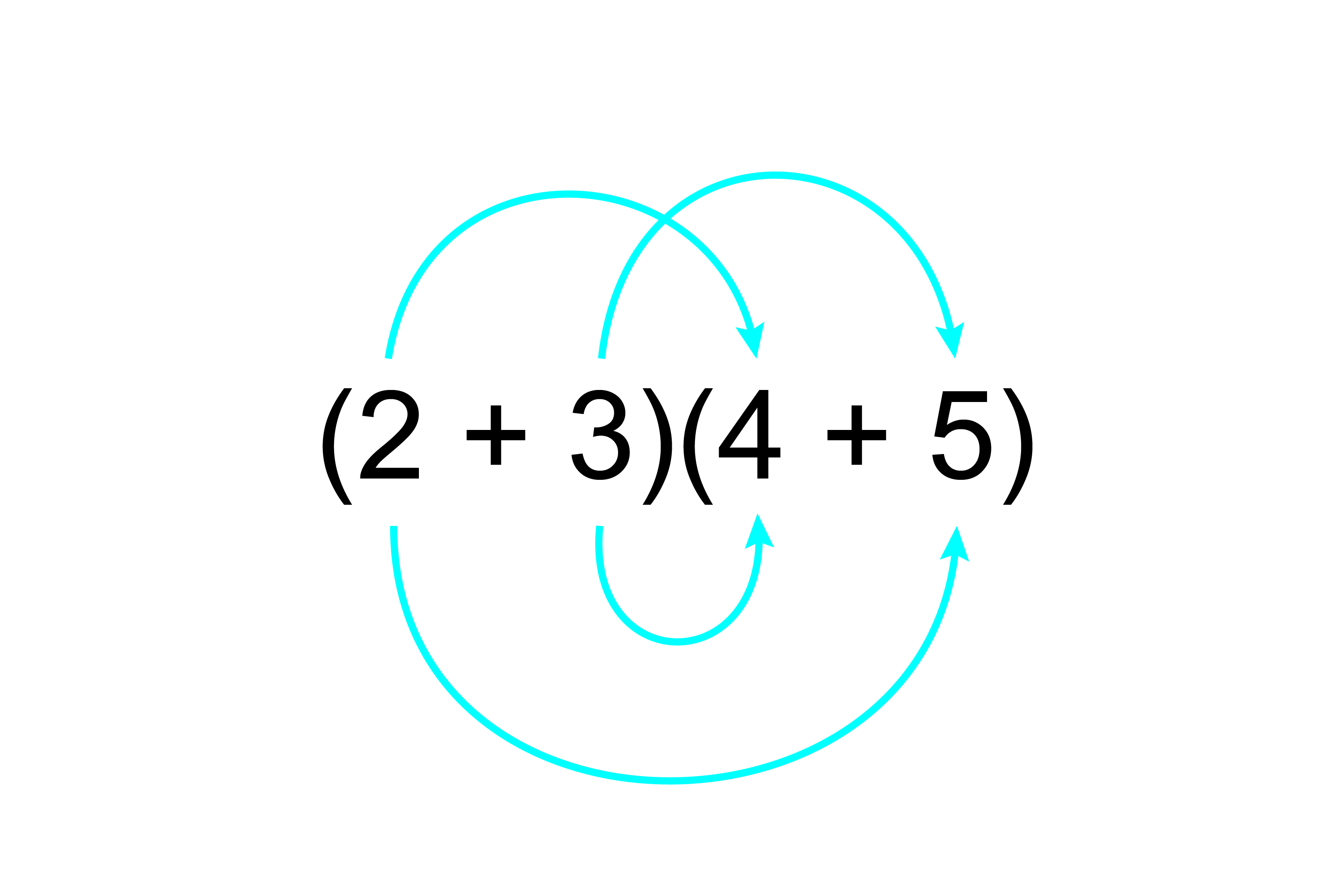The woman with the big nose.

2times4+2xx5+3xx4+3xx5

=8+10+12+15

=45

## Method 3 – The box method

(2+3)(4+5)

### Stage 1

 4 5 2 3

Now multiply the rows with the columns.

### Stage 2

 4 5 2 8 10 3 12 15

Now take the numbers out of the boxes.

=8+10+12+15

=45

## Method 4 - A visual way to remember multiplying out brackets

(a+b)^2=a^2+2ab+b^2

Which can be visualised as follows: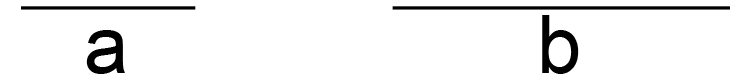Then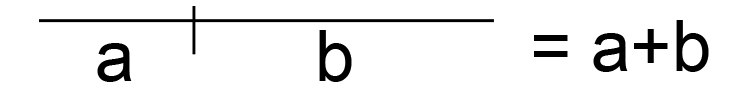But we want a+b squared

So lets make a square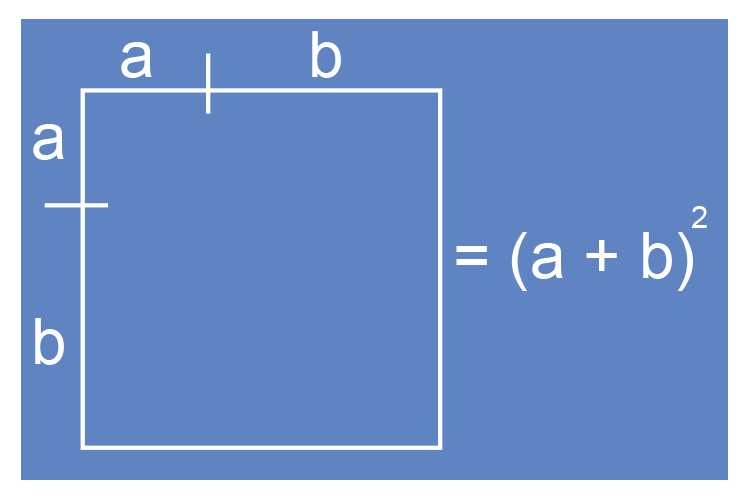Now fill in the box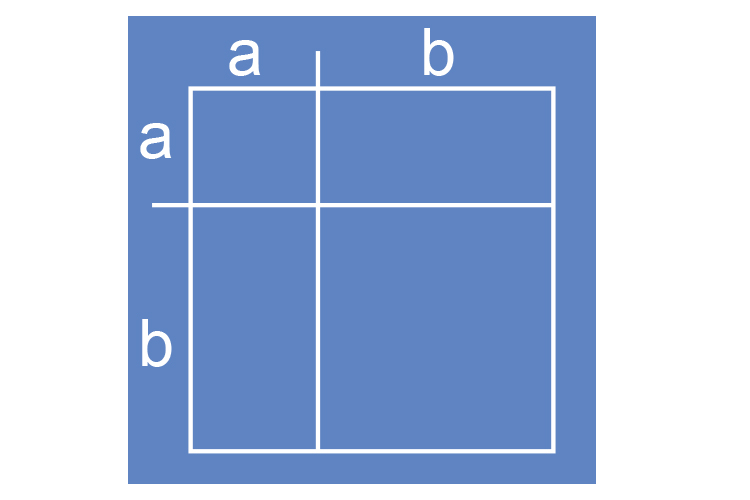The area of each square is: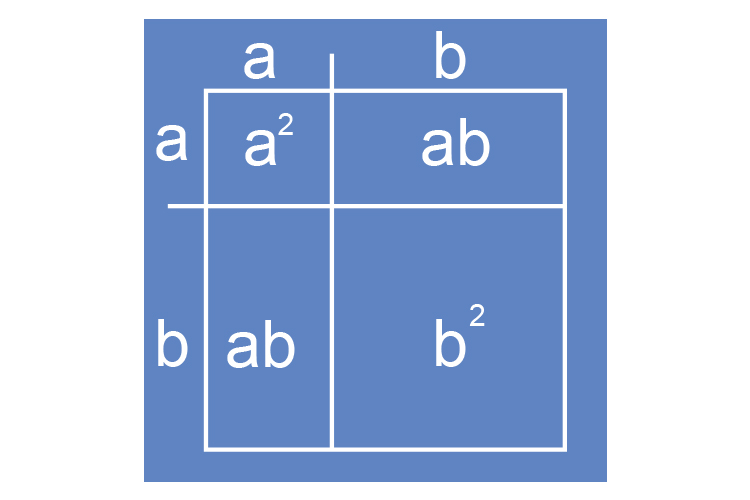So the area of this square is a^2+ab+ab+b^2

Which equals

a^2+2ab+b^2

So that's why

(a+b)^2=a^2+2ab+b^2

Examples

All four methods work but we will use method 1

1.  Multiply out (a+b)(c+d)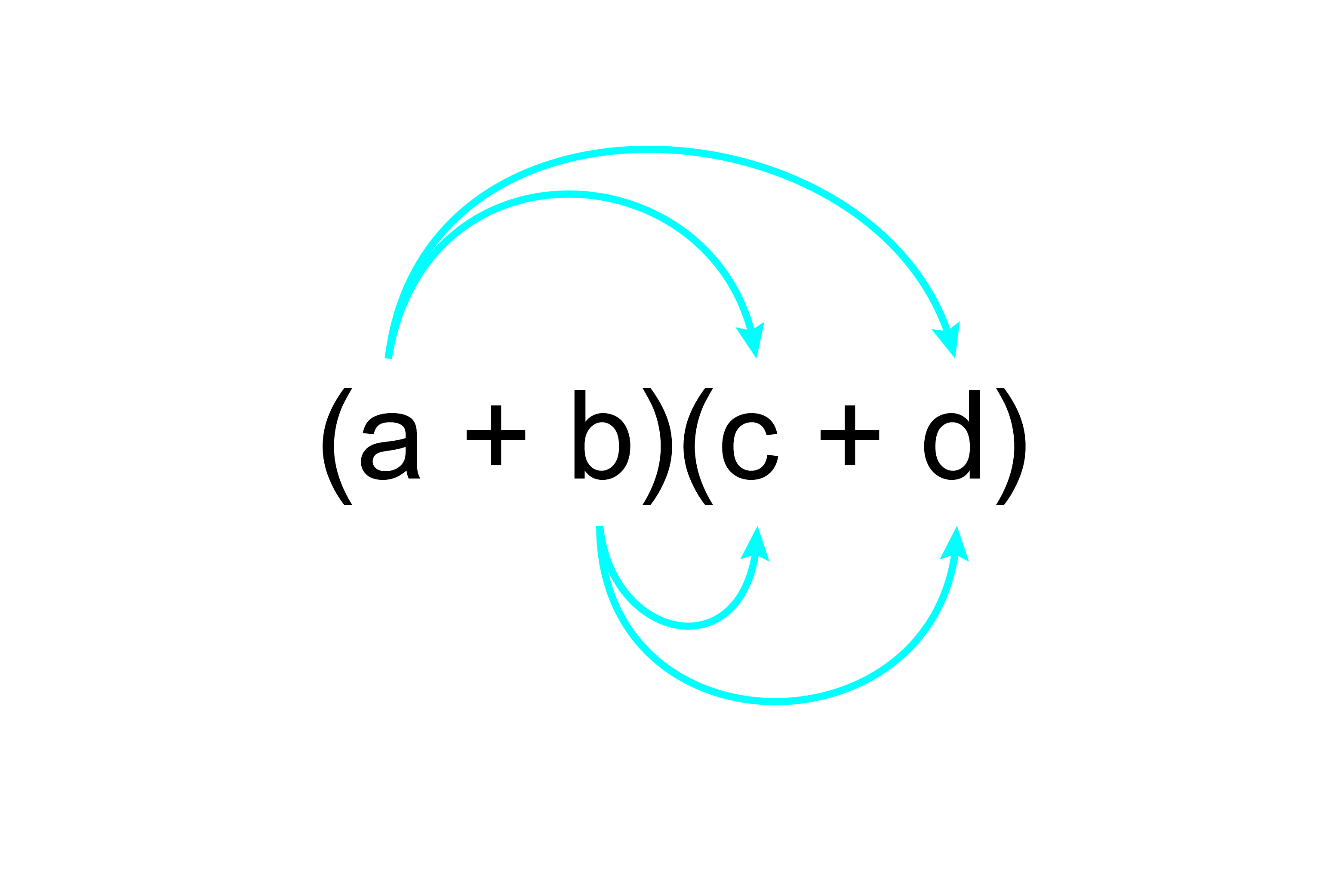ac+ad+bc+bd

Answer: (a+b)(c+d)=ac+ad+bc+bd

2.  Multiply out (5x+4y)(3x-4y)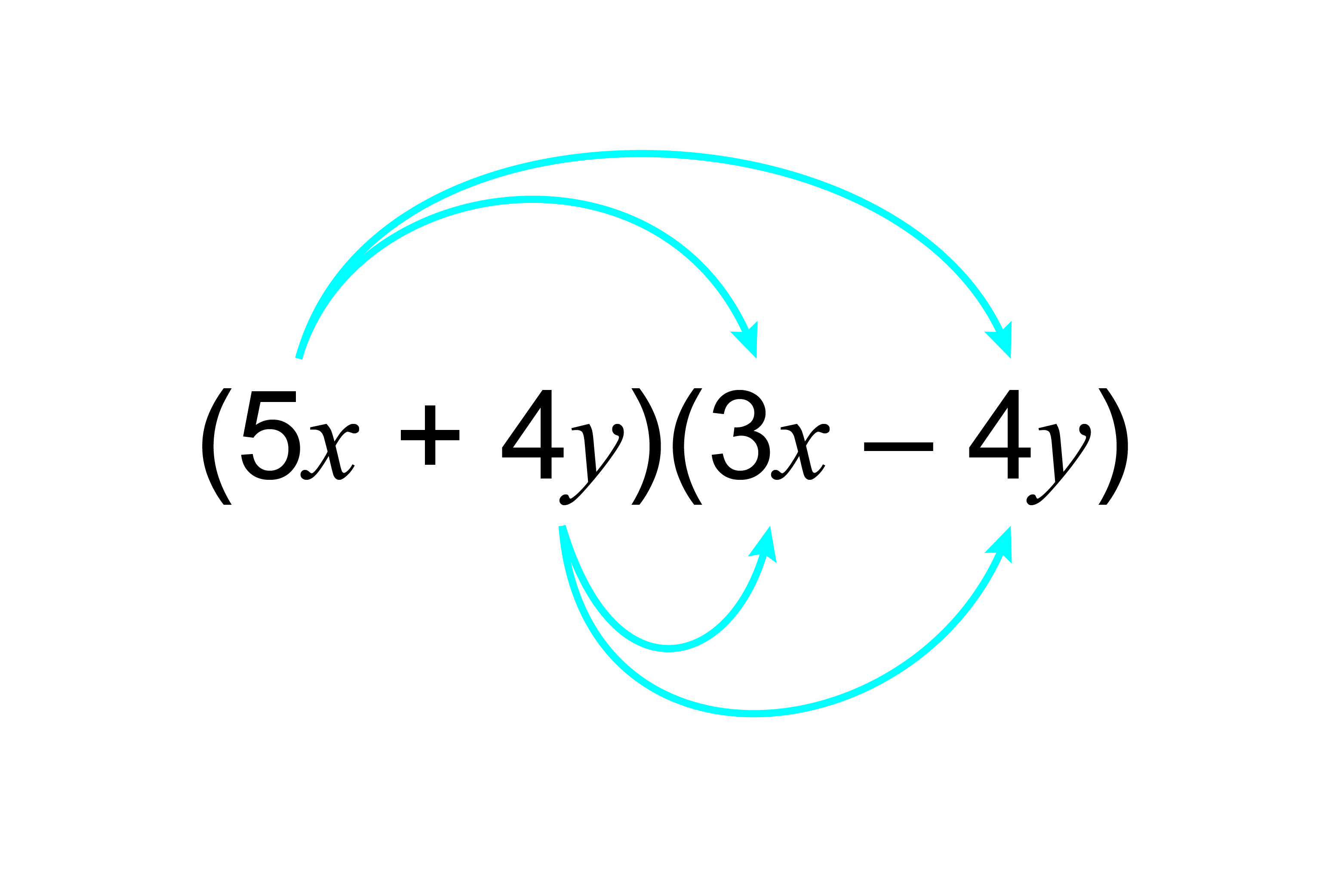5xtimes3x+5x(-4y)+4ytimes3x+4y(-4y)

15x-20xy+12xy-16y^2

15x-8xy-16y^2

Answer: (5x+4y)(3x-4y)=15x-8xy-16y^2

3.  Multiply out (x+8)^2

This is the same as (x+8)(x+8)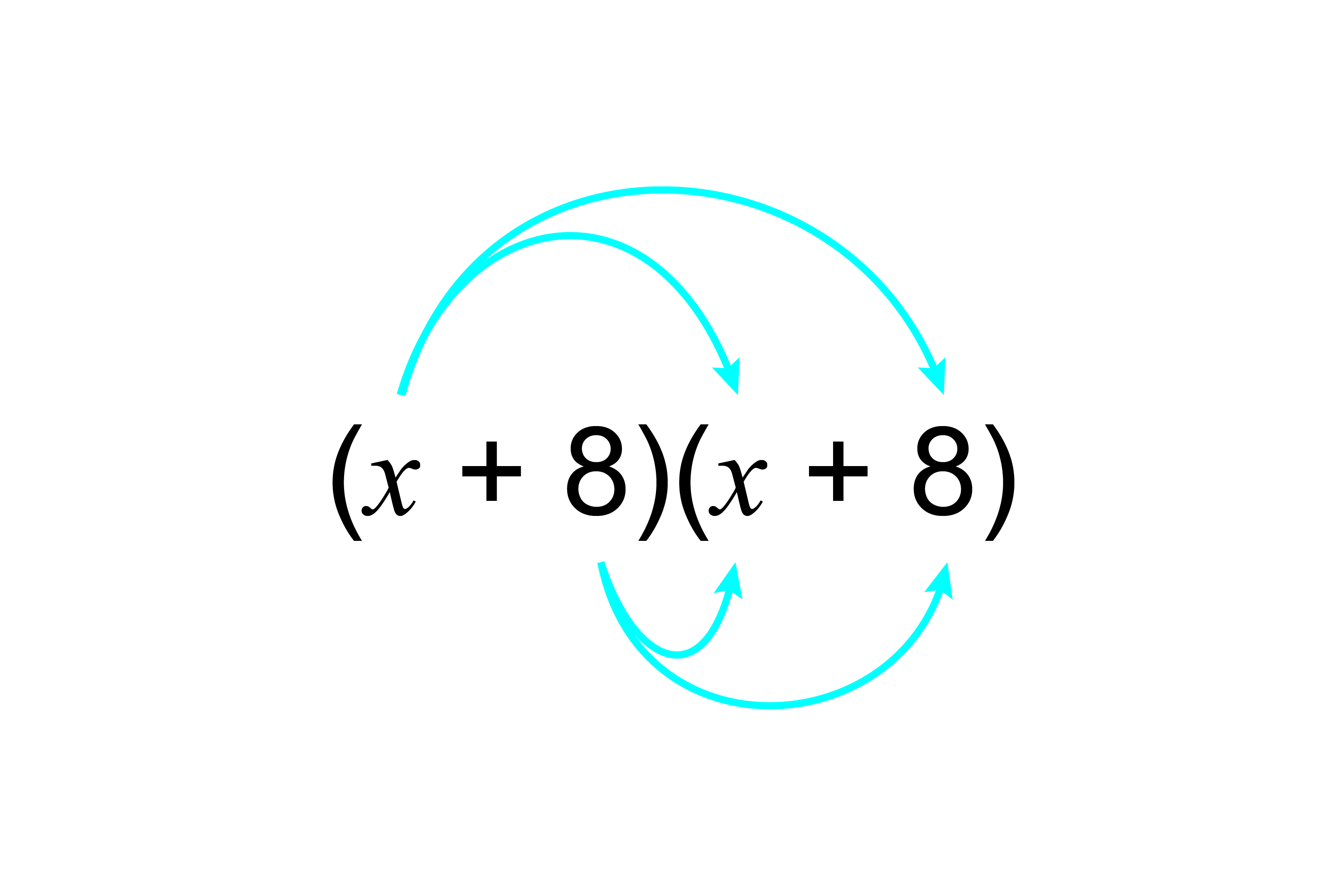x^2+8x+8x+8times8

x^2+16x+64

Answer: (x+8)^2=x^2+16x+64

4.  Multiply out 3(4x-7)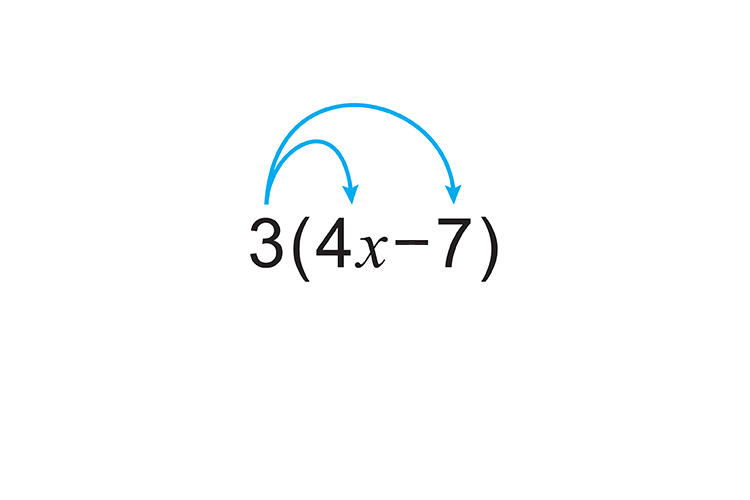3times4x-3times7

12x-21

Answer: 3(4x-7)=12x-21

5.  Multiply out 3xy(2x+y^2)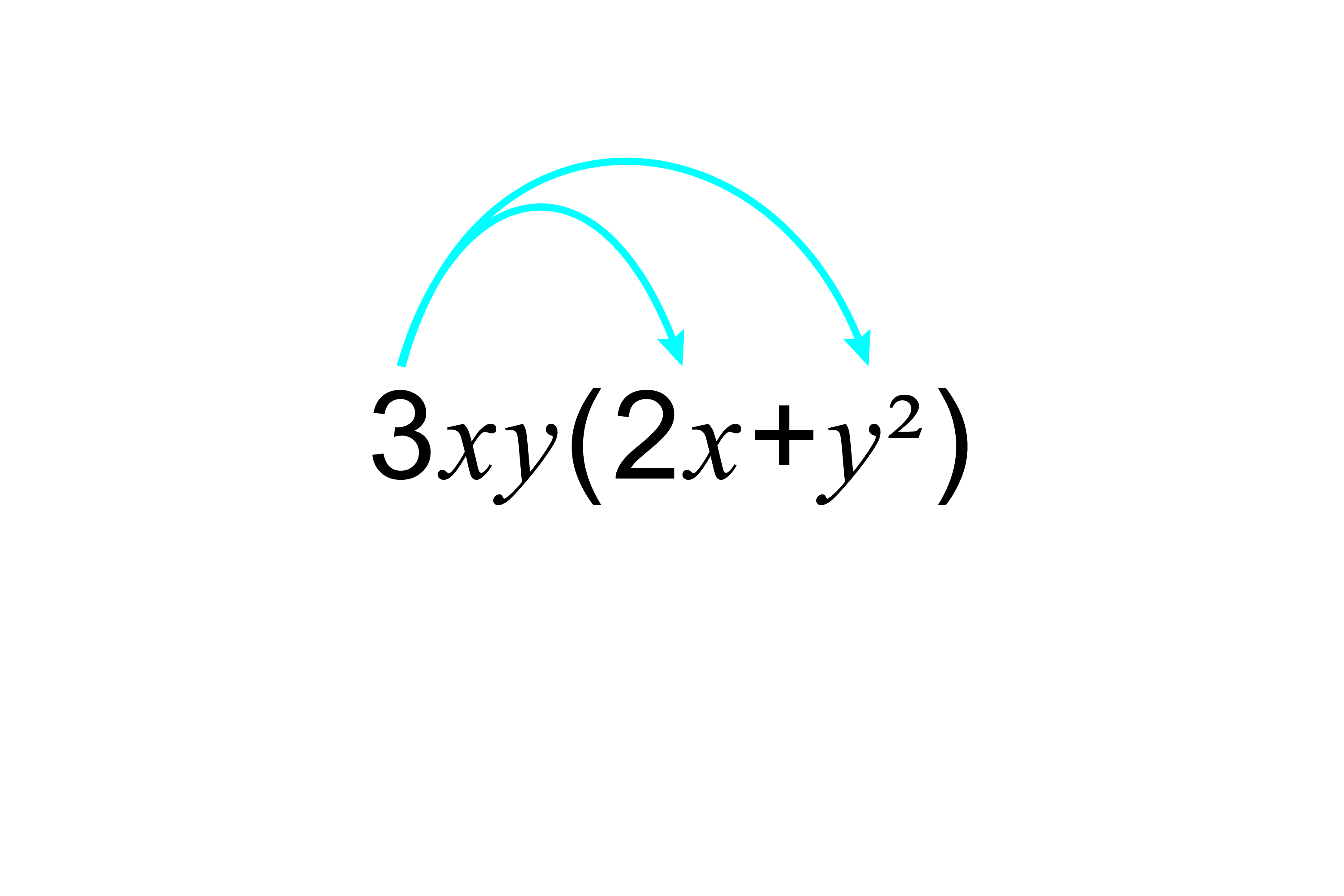3xytimes2x+3xytimesy^2

3timesxtimesytimes2timesx+3timesxtimesytimesytimesy

6x^2y+3xy^3

Answer: 3xy(2x+y^2)=6x^2y+3xy^3